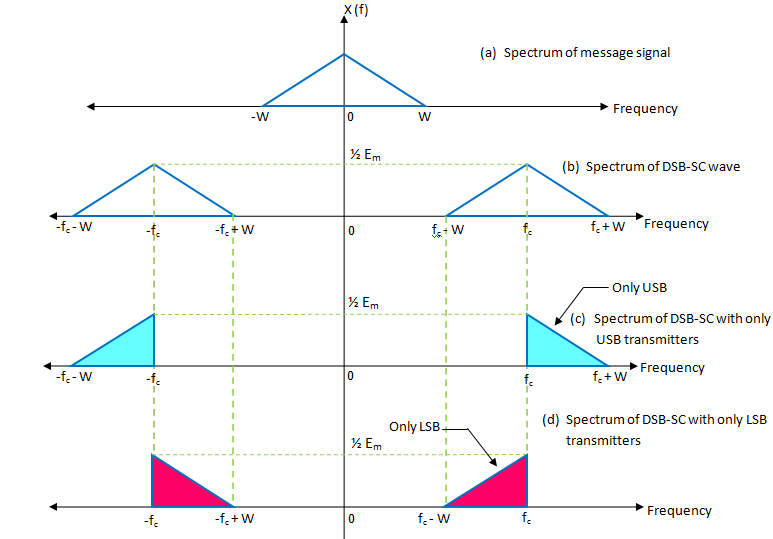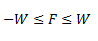# Single SideBand Suppressed Carrier (SSB-SC) Modulation

## Single SideBand Suppressed Carrier (SSB-SC) Modulation

As we know, the transmission bandwidth of standard AM as well as DSB-SC modulated wave is 2W Hz i.e., twice the message bandwidth W. Therefore, both these systems are bandwidth inefficient systems .

In both these systems, one half of the transmission bandwidth is occupied by the upper sideband (USB) and the other half is occupied by the lower sideband (LSB ) as shown in fig.1  .Fig 1

However, the information contained in the USB is exactly identical to that carried by the LSB . So, by transmitting both the sidebands we are transmitting the same information twice .

Hence, we can transmit only one sideband (USB or LSB ) without any loss of information . So, it is possible to suppress the carrier and one sideband completely .

When only one sideband is transmitted, the modulation is called as single sideband modulation . It is also known as SSB or SSB-SC modulation .

### Frequency Domain Description

Fig.1 (a) represents the spectrum X(f) of the message signal x(t) .

This spectrum is limited to the band :The spectrum of DSB-SC wave which is obtained by taking the product of x(t) and c(t) is shown in fig.1 (b) . It contains the USB as well as LSB .

When only USB is transmitted by the SSB system, then the corresponding spectrum is as shown in fig.1 (c) . And when only LSB is transmitted, the frequency spectrum is as shown in fig. 1 (d) .

Thus, the essential function of SSB modulation is to translate the spectrum of the message signal to a new location in the frequency domain .

### Transmission Bandwidth of SSB-SC

Since we are transmitting the frequencies only in the range (fc+ W) or ( fc – W), the transmission bandwidth for the SSB-SC will be :

Bandwidth B = (fc+ W) – f= W Hz

Or                 B= f–  ( fc – W) =W Hz

This is exactly half the bandwidth of the DSB-SC modulated waves .

### Advantages of SSB-SC Modulation Over DSB-FC

The advantages of SSB-SC over DSB-FC signal are :

1. Less bandwidth requirements . This allow more number of signals to be transmitted in the same frequency range .
2. Lots of power saving . This is due to the transmission of only one sideband component .At 100% modulation, the percent power saving is 83.33% .
3. Reduced interference of noise . This is due to the reduced bandwidth . As the bandwidth increases, the amount of noise added to the signal will increase .